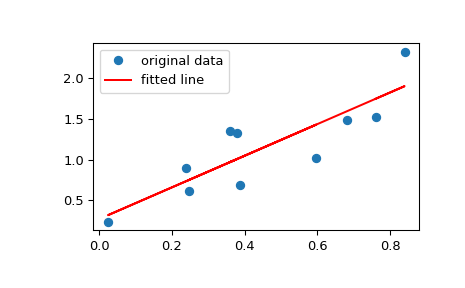# scipy.stats.linregress¶

scipy.stats.linregress(x, y=None)[source]

Calculate a linear least-squares regression for two sets of measurements.

Parameters
x, yarray_like

Two sets of measurements. Both arrays should have the same length. If only x is given (and y=None), then it must be a two-dimensional array where one dimension has length 2. The two sets of measurements are then found by splitting the array along the length-2 dimension. In the case where y=None and x is a 2x2 array, linregress(x) is equivalent to linregress(x, x).

Returns
slopefloat

Slope of the regression line.

interceptfloat

Intercept of the regression line.

rvaluefloat

Correlation coefficient.

pvaluefloat

Two-sided p-value for a hypothesis test whose null hypothesis is that the slope is zero, using Wald Test with t-distribution of the test statistic.

stderrfloat

Standard error of the estimated gradient.

scipy.optimize.curve_fit

Use non-linear least squares to fit a function to data.

scipy.optimize.leastsq

Minimize the sum of squares of a set of equations.

Notes

Missing values are considered pair-wise: if a value is missing in x, the corresponding value in y is masked.

Examples

>>> import matplotlib.pyplot as plt
>>> from scipy import stats


Generate some data:

>>> np.random.seed(12345678)
>>> x = np.random.random(10)
>>> y = 1.6*x + np.random.random(10)


Perform the linear regression:

>>> slope, intercept, r_value, p_value, std_err = stats.linregress(x, y)
>>> print("slope: %f    intercept: %f" % (slope, intercept))
slope: 1.944864    intercept: 0.268578


To get coefficient of determination (R-squared):

>>> print("R-squared: %f" % r_value**2)
R-squared: 0.735498


Plot the data along with the fitted line:

>>> plt.plot(x, y, 'o', label='original data')
>>> plt.plot(x, intercept + slope*x, 'r', label='fitted line')
>>> plt.legend()
>>> plt.show()Example for the case where only x is provided as a 2x2 array:

>>> x = np.array([[0, 1], [0, 2]])
>>> r = stats.linregress(x)
>>> r.slope, r.intercept
(2.0, 0.0)


#### Previous topic

scipy.stats.weightedtau

#### Next topic

scipy.stats.siegelslopes